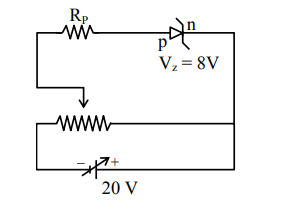# A zener diode having zener voltageQuestion:

A zener diode having zener voltage $8 \mathrm{~V}$ and power dissipation rating of $0.5 \mathrm{~W}$ is connected across a potential divider arranged with maximum potential drop across zener diode is as shown in the diagram. The value of protective resistance $R_{p}$ is............$\Omega$Solution:

$\mathrm{P}=\mathrm{Vi}$

$0.5=8 \mathrm{i}$

$\mathrm{i}=\frac{1}{16} \mathrm{~A}$

$E=20=8+i R_{P}$

$\mathrm{R}_{\mathrm{P}}=12 \times 16=192 \Omega$